﻿ Prediction of Young’s Modulus of Graphene Sheets by the Finite Element Method

### Prediction of Young’s Modulus of Graphene Sheets by the Finite Element Method

Pavol Lengvarský, Jozef Bocko

American Journal of Mechanical Engineering

## Prediction of Young’s Modulus of Graphene Sheets by the Finite Element Method

Pavol Lengvarský1,, Jozef Bocko1

1Department of Applied Mechanics and Mechanical Engineering, Faculty of Mechanical Engineering, Technical University of Košice, 042 00 Košice, Slovak Republic

### Abstract

Young’s moduli of graphene sheets are investigated in this paper. Graphene sheet is modelled as space frame structure by beam elements. Properties of beam elements are obtained from linkage molecular mechanics and classic continuum mechanics. Interatomic interactions in molecular mechanics are assigned to structural mechanics, and then diameter and elastic properties for beam elements are obtained. Graphene sheet is modelled with commercial finite element code and the boundary conditions are applied in two different directions. Young’s moduli of graphene sheets vary with dimensions of sheets and they are affected by direction of loading. Finally, the Poisson’s ratios are obtained from deformations of graphene sheets.

• Pavol Lengvarský, Jozef Bocko. Prediction of Young’s Modulus of Graphene Sheets by the Finite Element Method. American Journal of Mechanical Engineering. Vol. 3, No. 6, 2015, pp 225-229. http://pubs.sciepub.com/ajme/3/6/14
• Lengvarský, Pavol, and Jozef Bocko. "Prediction of Young’s Modulus of Graphene Sheets by the Finite Element Method." American Journal of Mechanical Engineering 3.6 (2015): 225-229.
• Lengvarský, P. , & Bocko, J. (2015). Prediction of Young’s Modulus of Graphene Sheets by the Finite Element Method. American Journal of Mechanical Engineering, 3(6), 225-229.
• Lengvarský, Pavol, and Jozef Bocko. "Prediction of Young’s Modulus of Graphene Sheets by the Finite Element Method." American Journal of Mechanical Engineering 3, no. 6 (2015): 225-229.

 Import into BibTeX Import into EndNote Import into RefMan Import into RefWorks

1234
Prev Next

### 1. Introduction

Graphene (Figure 1) is a two-dimensional hexagonal (honeycomb) lattice made of carbon atoms which size is in nanometres. Nowadays, materials in nanometres are widely investigated for their extraordinary properties. Researches study their mechanical properties and their applications in classic materials. Young’s modulus, tensile or compressive strength and buckling are investigated by number of researchers. Intensive research is also oriented to the application of nanostructures in electrical and chemical engineering, as well as in biological sciences. Nanomaterials are investigated with two approaches i.e. the molecular mechanics approach and the continuum mechanics approach [1-12].Download asVeiw figureFigures index
Figure 1. Graphene

Practical application of graphene is in advanced composites, nano-electro-mechanical-systems (NEMS) and electronics, because it has high mechanical properties and low mass destiny .

### 2. Continuum Mechanics Approach

Young’s modulus is calculated from deformation of graphene sheet but at the beginning the knowledge of beam element parameters is necessary. For this sake we define connection between molecular mechanics and continuum mechanics [2, 3, 5].

In molecular mechanics graphene sheet can be regarded as a large molecule consisting of carbon atoms and the atomic nuclei as material points. Motions of atomic nuclei are regulated by a force field, which is generated by electron-nucleus interactions and nucleus-nucleus interaction and the force field is expressed in the form of steric potential energy . The total steric potential energy [4-10] omitting the electrostatic interaction, is a sum of energies due to valence or bonded interactions and non-bonded interactions are expressed as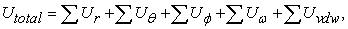(1)

where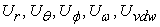are a bond stretch interaction, a bond angle bending, a dihedral angle torsion, an improper (out of plane) torsion, a non-bonded van der Waals interaction, respectively. Representations of these interactions are given in Figure 2 [6, 11, 12].

The main contributions to the total steric energy come from the first four terms of equation (1). Under the assumption of small deformation, the harmonic approximation is adequate for describing the energy . By adopting the simplest harmonic forms and merging dihedral angle torsion and out-of-plane torsion into a single equivalent term, we can write relations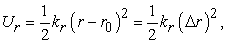(2)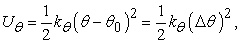(3)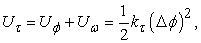(4)

where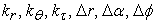are the bond stretching force constant, bond angle bending force constant, torsional resistance, the bond stretching increment, the bond angle change and the angle change of bond twisting, respectively [2, 3].Download asVeiw figureFigures index
Figure 2. Interatomic interactions in molecular mechanics 

To calculate the elastic moduli of beam elements we determine relations between the sectional stiffness parameters in structural mechanics and the force constants in molecular mechanics.Download asVeiw figureFigures index
Figure 3. Tension, bending and torsion of an element 

The sections of beams which represent carbon-carbon bonds are assumed as identical, circular and moments of inertia are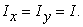Than we get three stiffness parameters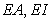and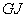from linkage among the energy terms in molecular mechanics and continuum mechanics as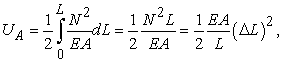(5)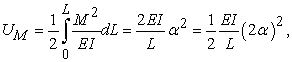(6)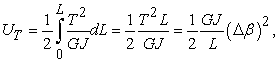(7)

where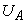is the strain energy of a uniform beam of length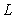subjected to axial force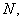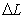is axial elongation,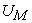is the strain energy of a uniform beam under bending moment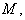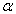is the rotational angle at the ends of the beam,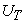is the strain energy of a uniform beam under tension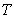and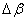is the relative rotation between the ends of the beam (Figure 3) [2, 3].Download asVeiw figureFigures index
Figure 4. Interatomic interactions in molecular 

Thus by comparing equations (2)-(4) and (5)-(7) we get terms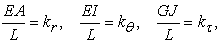(8)

Then beam element (Figure 4) is adopted and its elastic properties for further analysis are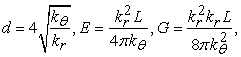(9)

where constants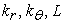are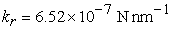,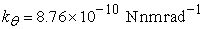and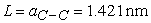.

Terms in (9) are calculated and we get diameter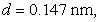elastic moduli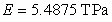and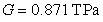for beam members.

### 3. Finite Element Simulation of Graphene Sheet

The numerical computations were accomplished by commercial program Ansys. Graphene sheet is modelled as frame structure by beam elements which have had parameters mentioned above. Carbon atoms are considered to be nodes in structure and the nodes are joined by beam elements. The structure is fully restrained on one side and loaded by tensile force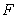on other side. The finite element analysis was performed for two different boundary conditions (graphene sheet rotated by 90°) as is shown in Figure 5 and Figure 6.Download asVeiw figureFigures indexDownload asVeiw figureFigures index

From the computation we get the deformations of graphene sheet and accordingly its elongation (Figure 7).

### 4. Young’s Modulus of Graphene Sheet

The Young’s modulus is ratio of normal stress to normal strain obtained from equation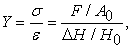(10)

where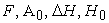are total force applied on graphene sheet, the cross-section of graphene sheet, the elongation of graphene sheet and the initial length of graphene sheet, respectively.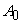is equal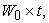where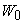is the initial width of graphene sheet and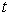is the thickness of graphene sheet i.e.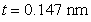. Poisson’s ratio of graphene sheet is calculated from equation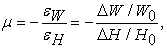(11)

where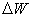is constriction of graphene sheet.Download asVeiw figureFigures index
Figure 7. Deformation of graphene sheet

Then Young’s moduli and Poisson’s ratios were computed and some results are given in Table 1.

#### Table 1. Young’s moduli for different dimensions of graphene sheets

All computed Young’s moduli are shown in Figure 8. It is clear that Young’s moduli of graphene sheets are significantly affected by the size of width of graphene sheet and by direction of loading. Young’s modulus varies slightly with increasing length of graphene sheet and boundary conditions also slightly affect magnitude of Young’s modulus of graphene sheet.Download asVeiw figureFigures index
Figure 8. Variation Young’s modulus with variation length of graphene sheet

Poisson’s ratio for graphene sheet is computed from equation (11) and corresponding charts are shown in Figure 9. From the results is apparent that only direction of loading markedly affects magnitude of Poisson’s ratio.Download asVeiw figureFigures index
Figure 9. Variation Poisson’s ratio with variation length of graphene sheet

#### Table 2. Young’s moduli for different dimensions of graphene sheets

Some authors use in equation (10) in termforvalue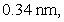which is the interlayer spacing of graphite. In Table 2 are given new values of Young’s moduli of graphene sheet and corresponding dependencies. Young’s modulus is affected with dimensions and direction of loading of graphene sheet too, but the influence is smaller than in previous case (Figure 10).Download asVeiw figureFigures index
Figure 10. Variation Young’s modulus with variation length of graphene sheet

### 5. Conclusion

A linkage between molecular mechanics and structural mechanics has been proposed. Space frame structure was simulated by this linkage. For the computation was used finite element model with beam elements of proposed diameter and elastic properties (Young’s moduli). This model is based only on beam elements that represent C-C bonds and joints that represent carbon atoms. Beam element has two nodes with the six degrees of freedom at each node. The beam connects individual atoms (joints). Analysis has been performed on graphene sheets with different dimensions like width, length and with two different loading directions. The same boundary conditions were applied consecutively to two mutually perpendicular directions.

This model was used for evaluation of Young’s modulus and Poisson’s ratio of graphene sheet. Young’s modulus is affected by dimensions, direction of loading of graphene sheet and Poisson’s ratio is affected by direction of loading of graphene sheet. Young’s modulus and Poisson’s ratio increase slightly with increasing length of graphene sheet. Magnitudes of Young’s modulus and Poisson’s ratios are comparable with results published by several researchers from theoretical, numerical (molecular, structural simulations) and experimental measurements.

### Acknowledgement

This article was created with support of VEGA grant project VEGA 1/1205/12 Numerical modelling of mechatronic systems.

### References

  Marenić, E., Ibrahimbegovic, A., Sorić, J. Guidault, P.A., “Homogenized elastic properties of graphene for small deformations,” Materials, 6. 3764-3782. 2013.In article View Article  Li, Ch., Chou,T. “A structural mechanics approach for the analysis of carbon nanotubes,” International Journal of Solids and Structures, 40. 2487-2499. Jan.2003.In article View Article  Tserpes, K.I., Papanikos, P., “Finite element modelling of single-walled carbon nanotubes,” Composites Part B, 36. 468-477. 2005.In article View Article  Machida, K., Principles of Molecular Mechanics, Kodansha and John Wiley & Sons Co-publication, Tokyo. 1999.In article  Tertel, E., Kurylo, P., Papacz, W., “The stress state in the three-layer open conical shell during of stability loss,” Acta Mechanica Slovaca, 18 (2). 56-63. Aug.2014.In article  Mayo, S.L., Olafson, B.D., Goddard, W.A., “Dreiding–a generic force-field for molecular simulations,” Journal of Physical Chemistry, 94. 8897-8909. 1990.In article View Article  Cornell, W.D., Cieplak, P., Bayly, C.I., et al., “A second generation force-field for the simulation of proteins, nucleic-acids, and organic-molecules,” Journal of American Chemical Society 117. 5179-5197. 1995.In article View Article  Brenner, D.W., “Empirical potential for hydrocarbons for use in simulating the chemical vapor deposition of diamond films,” Physical Review B, 42. 9458. 1990In article View Article  Rappe, A.K., Casewit, C.J., Colwell, K.S., et al., “A full periodic-table force-field for molecular mechanics and molecular dynamics simulations,” Journal of American Chemical Society, 114. 10024-10035. 1992.In article View Article  Ru, C.Q., “Effective bending stiffness of carbon nanotubes,” Phys Rev B, 62. 9973-9976. 2000In article View Article  Saito, S., Dresselhaus, D., Dresselhaus, M.S., Physical Properties of Carbon Nanotubes, Imperical College Press, London. 1998In article  Thostenson, E.T., Chunyu, L., Chou, T.W. “Nanocomposites in context,” Composite Science and Technology, 65. 491-516. 2005.In article View Article